# Introduction

During the model estimation proces, rtrim also computes model parameter uncertainties, expressed as variances and standard errors, which are propagated into standard errors for final model-derived statistics like time-totals and indices. To assist comparison with other trend analysis methods, it might be helpful if the rtrim output uncertainties can be presented as confidence intervals as well. In this document, we present a method to convert rtrim standard errors into, say, 95% coinfidence intervals. Given the particular distributions that are commonly used to model count data (Poisson, negative binomial), the standard approach of multiplying standard errors with a constant factor to obtain a confidence interval will not work, and an alternative approach will be developed.

rm(list=ls()) # Always start with a clean slate
library(rtrim)
red   <- "#E41A1C" # Set up some nice colors
blue  <- "#377EB8"
green <- "#4daf4a"
lgray <- gray(0.8)
mgray <- gray(0.5)
dgray <- gray(0.2)

# Example: normal distribution

For a normal distribution with mean $$\mu$$ and standard deviation $$\sigma$$, it is well known that the 95% confidence interval corresponds to $$\mu\pm1.96\sigma$$, as illustrated here:

mu    <- 5.0                            # mean
sd    <- 2.0                            # standard deviation
alpha <- 0.05                           # i.e., 95% confidence interval

# Full normal distribution
x <- seq(mu-3*sd, mu+3*sd, len=100)
y <- dnorm(x, mean=mu, sd=sd)

# Use quantile function to compute the confidence interval (CI)
lo <- qnorm(alpha/2,   mean=mu, sd=sd)  # lower CI bound
hi <- qnorm(1-alpha/2, mean=mu, sd=sd)  # upper CI bound

plot(NULL,NULL, type='n', xlim=range(x), ylim=range(y),
xlab=NA, ylab="Probability density", las=1)

xci <- seq(lo, hi, len=100)             # background: confidence interval
yci <- dnorm(xci, mean=mu, sd=sd)
xx <- c(xci, rev(xci))
yy <- c(0*yci, rev(yci))
polygon(xx,yy,col=gray(0.9), border=NA)

lines(x,y, col=red, lwd=2)              # Foreground: complete distribution

# Annotation and decoration
lines(c(mu,mu), c(0,dnorm(mu,mean=mu,sd=sd)), lty="dashed", lwd=0.5) # mean
lines(c(mu-sd,mu-sd), c(0,dnorm(mu-sd,mean=mu,sd=sd)), lty="dashed", lwd=0.5) # mu - s.d.
lines(c(mu+sd,mu+sd), c(0,dnorm(mu+sd,mean=mu,sd=sd)), lty="dashed", lwd=0.5) # mu + s.d.
abline(h=0, lwd=0.5) # proper y=0 line
text(mean(x), mean(y), sprintf("%.0f%%", 100*(1-alpha)))
yarr <- 0.02                            # y-position of arrow
arrows(mu-sd,yarr, mu,yarr, code=3,length=0.12)
text(mu-sd/2, yarr, "s.d.", pos=3)
mul <- (hi-mu) / sd                     # sd -> CI multiplier
arrows(mu,yarr, hi,yarr, code=3, length=0.12)
text(mean(c(mu,hi)), yarr, sprintf("%.2f * s.d.", mul), pos=3)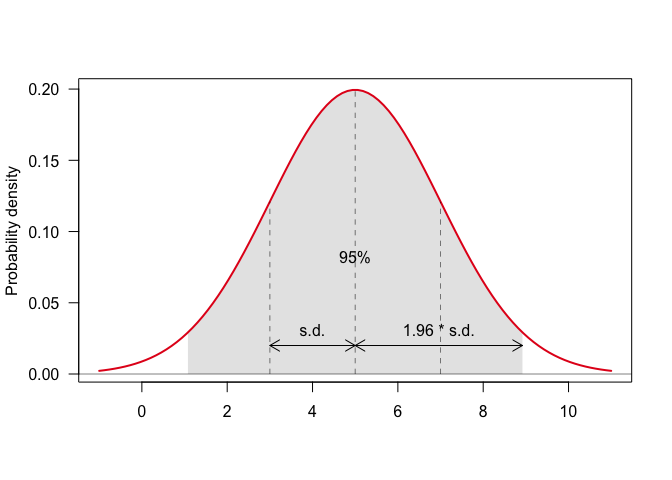Note that the confidence interval of the distribution is found using the so-called quantile function, which is the inverse of the cumulative distribution.

Here is a graphical display of the relation between the inverse of the cumulative distribution and the said multiplication factor:

mu <- 0                                 # Standard normal distribution
sd <- 1.0
alpha <- 0.05                           # 95% confidence interval
xcdf <- seq(mu-3*sd, mu+3*sd, len=100)  # cumulative distribution function
ycdf <- pnorm(xcdf, mean=mu, sd=sd)
plot(NULL,NULL, xlim=range(xcdf), ylim=c(0,1),
xlab="Value", ylab="Cumulative distribution function", las=1)
# connect mu with median
x0 <- min(xcdf)
x0 <- -2.8
pp <- c(alpha/2, 0.5, 1-alpha/2)
for (i in 1:length(pp)) {
p <- pp[i]
x <- qnorm(p, mean=mu, sd=sd)
y0 <- ifelse(i==3, 0.04, 0)
lines(c(x0, x,x), c(p,p,y0), col=mgray)
text(-3,p,sprintf("%.3f", p), cex=0.8, col=mgray)
if (i==3) text(x,0, sprintf("%.2f", x), cex=0.8, col=mgray)
xmid <- (x0+x)/2
arrows(xmid,p,xmid+0.01,p, col=mgray, length=0.1)
if (p<0.1) next # skip vertical arrows if there is no place
arrows(x,p/2,x,p/2-0.01, col=mgray, length=0.1)
}
# Foreground: CDF
lines(xcdf,ycdf, col=red, lwd=2)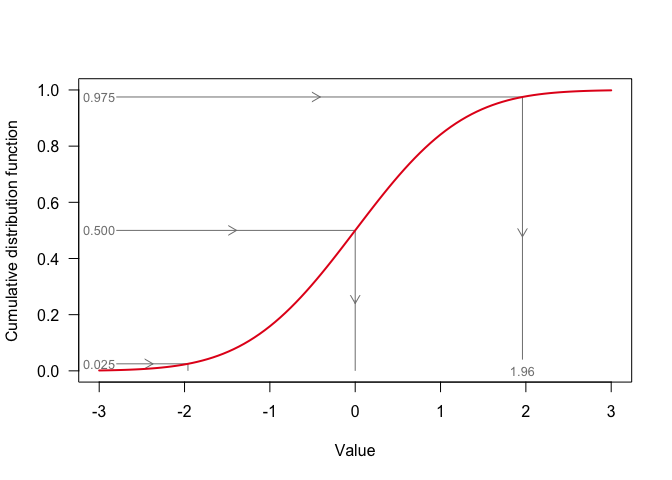# TRIM without overdispersion: Poisson distribution

In TRIM, one of the basic assumptions is that observations, which are counts, are assumed to be Possion distributed, with probability mass function (PMF) $f(k; \lambda) = \frac{\lambda^k e^{-k}}{k!}$ where $$k\in\mathbb{N}$$ is the count level, and $$\lambda$$ is rate parameter.

For this discrete distribution, the first two moments, i.e. the expected value $$\operatorname{E}(k)$$ and variance $$\operatorname{var}(k)$$, are given by $\operatorname{E}[k]=\lambda \quad\text{and}\quad \operatorname{var}[k]=\lambda$

Here is an example for $$\lambda=5$$, plotting the cumulative distribution function (CDF) $F(k; \lambda) = \frac{\Gamma(\lfloor k+1\rfloor,\lambda)}{\lfloor k\rfloor!}$

lambda <- 5L # Expected value
x <- 0 : (lambda*3)
y <- ppois(x, lambda)
plot(x, y, type='n', xlab="Value", ylab="Cumulative distribution function", las=1) # empty
# background: indicate expected value
abline(v=lambda, col=mgray, lty="dashed")
text(lambda, 0.1, expression(lambda), col=mgray, pos=4)
# foreground on top
lines(x, y, type='s', col=red)
points(x,y, pch=16, col=red)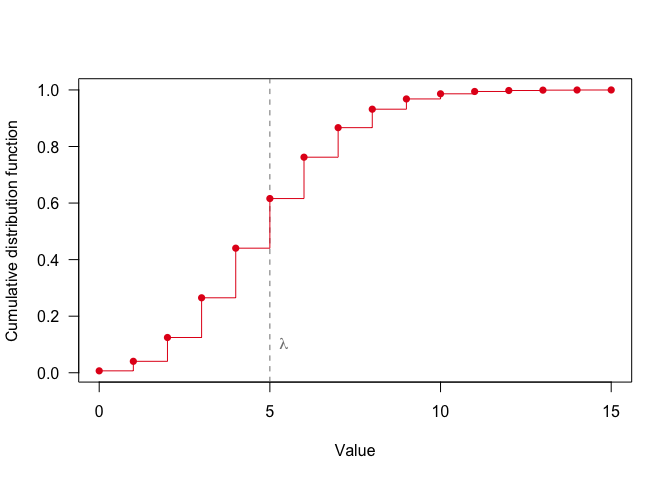The problem with the Poisson distribution in this context is it’s discrete nature: the CDF is discontinuous, so a given confidence level of, say, 95% is not uniquely related to corresponding standard errors of counts.

In practice, the R function qpois() that implements the inverse CDF returns a quantile for any probability, with discrete jumps:

lambda <- 5L # Expected value
x <- 0 : (lambda*3)
y <- ppois(x, lambda)
plot(x, y, type='n', xlab="Value", ylab="Cumulative distribution function", las=1)
# background: indicate discrete cdf->quantile
cdf_to_quantile <- function(p, ...) {
q <- qpois(p, ...)
xx <- c(0,q,q)
yy <- c(p,p,0)
lines(xx,yy, col=mgray)
arrows(q/2,p,q/2+0.01,p, length=0.1, col=mgray) # add arrow
arrows(q,p/2,q,p/2-0.01, length=0.1, col=mgray)
}
cdf_to_quantile(0.74, lambda=lambda)
cdf_to_quantile(0.78, lambda=lambda)
# cdf on top
lines(x, y, type='s', col=red)
points(x,y, pch=16, col=red)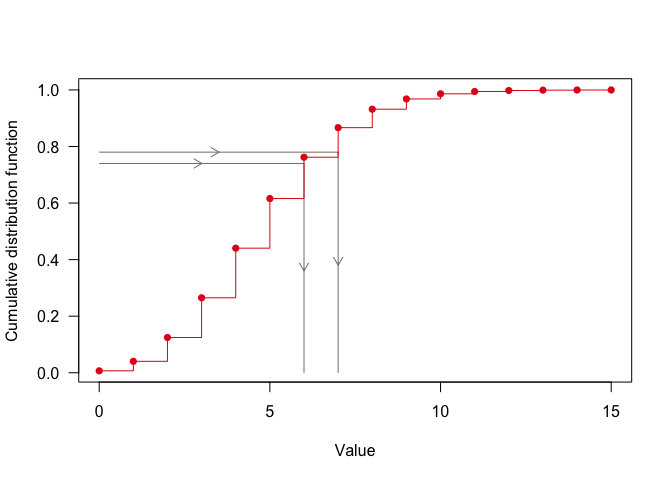In principle, one could use this discrete approach: use the qpois() function to compute the (discrete) quantiles, and use these in conjunction with the (discrete) expected value and variance (which are equal by definition) to compute the multipliers.

However, a couple of TRIM properties invalidate this approach for many use cases. For a true Poisson distributed variable $$x$$ the variance $$\operatorname{var}(x)$$ is always an integer, because it is identical to the expected value, which is integer: $$\operatorname{var}(x) \equiv \operatorname{E}(x) \in \mathbb{N}$$. TRIM, however, relaxes these requirements. First, count data does not necessarily have to be integer. For example, if the counts’ are the results of a prior aggregation process. Second, equivalence of variance and expected value is relaxed, allowing for $$\operatorname{var}(x) \propto \operatorname{E}(x)$$, i.e. overdispersion. Both arguments result in variances being continuous, therefore invalidating the discrete approach outlined above.

## Poisson-Gamma equivalence

The approach used in TRIM is to enforce continuity by approximating the discrete Poisson distribution by the continuous Gamma distribution, which probablity density function (PDF) and CDF are given by $f(x; k, \theta) = \frac{1}{\Gamma(k)\theta^k} x^{k-1} e^{-x/\theta}$ and $F(x; k, \theta) = \frac{1}{\Gamma(k)} \gamma\left(k,\frac{x}{\theta}\right)$ where $$k$$ and $$\theta$$ are a and parameter.

The first two moments are given by $\operatorname{E}[x]=k\theta \quad\text{and}\quad \operatorname{var}[x] = k \theta^2$

In order to fit the Gamma distribution on the Poisson distribution, we equate the first two moments: $k\theta=\lambda;\quad k\theta^2=\lambda \Longrightarrow k=\lambda;\quad \theta=1$ which suggest that a Poisson distribution with rate $$\lambda$$ can be approximated by a Gamma distribution with $$k=\lambda$$ and $$\theta=1$$.

Here is a test of this result:

# Plot Poisson distribution (expected value: 10)
lambda <- 10L # define expected value
xp <- 0 : (lambda * 3L)
yp <- ppois(xp, lambda=lambda)
plot(xp, yp, type='s', pch=16, col=red,
xlab="Value", ylab="Cumulative distribution function", las=1)
points(xp,yp, pch=16, col=red)

# Plot equivalent gamma
xg <- xp # same range
yg <- pgamma(xg, shape=lambda, scale=1)
lines(xg, yg, col=blue, lwd=2)

abline(v=lambda, lty="dashed", col=mgray) # mark expected value
text(lambda, 0.2, expression(lambda), pos=4, col=mgray)
legend(0,1, legend=c("Poisson","Gamma"), col=c(red,blue), lty="solid", lwd=2)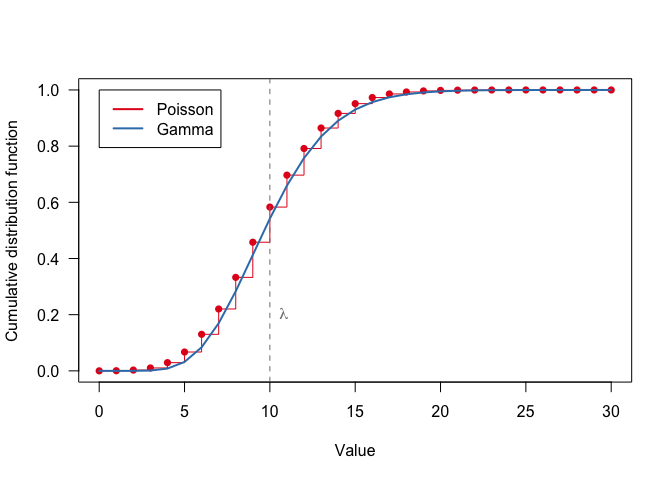As can be seen, the fit is, although not perfect, quite satisfactory since the Gamma curve remains wthin the Poisson ‘steps’.

The mismatch between Poisson and Gamma distibutions decreases rapidly for larger expected values. Here is an example for $$\lambda=50$$:

lambda <- 50L # define expected value
xp <- 0 : (lambda * 3L)
yp <- ppois(xp, lambda=lambda)
plot(xp, yp, type='s', pch=16, col=red,
xlab="Value", ylab="Cumulative distribution function", las=1)
points(xp,yp, pch=16, col=red)
# Plot equivalent gamma
xg <- xp # same range
yg <- pgamma(xg, shape=lambda, scale=1)
lines(xg, yg, col=blue, lwd=2)
abline(v=lambda, lty="dashed", col=mgray) # mark expected value
text(lambda, 0.2, expression(lambda), pos=4, col=mgray)
legend(0,1, legend=c("Poisson","Gamma"), col=c(red,blue), lty="solid", lwd=2)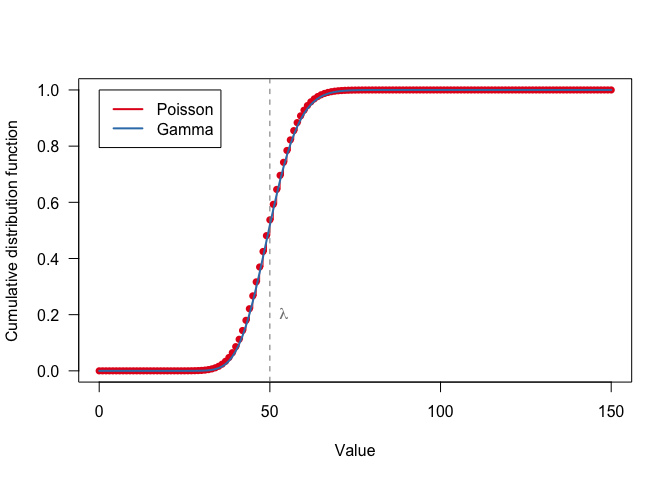This is perfectly acceptable.

## Multipliers

The next step is to compute the multipliers, i.e. the ratio between quantiles corresponding to the 95%, say, confidence interval bounds and the standard errors. Unlike the normal distribution, where this ratio is a constant (1.96), for the gamma distribution the ratio depends on the distribution parameter, i.e. expected value $$\lambda$$.

Denoting $$Q_{0.025}$$ and $$Q_{0.975}$$ for the lower and upper quantile of the 95% C.I., the corresponding multipliers $$M_{0.025}$$ and $$M_{0.975}$$ are computed as $M_{0.025} = \frac{|Q_{0.025}-\operatorname{E}(x)|}{\sqrt{\operatorname{var}(x)}} = \frac{|Q_{0.025} - \lambda|}{\sqrt{\lambda}}$ and $M_{0.975} = \frac{\left|Q_{0.975}-\operatorname{E}(x)\right|}{\sqrt{\operatorname{var}(x)}} = \frac{|Q_{0.975} - \lambda|}{\sqrt{\lambda}}$ where the absolute value of the numerator is taken to guarantee positive multipliers.

A graphical display of the depence of both multipliers on expected value is plotted below:

lambda <- exp(seq(log(2.0), log(1000.0), len=100)) # uniform spacing in log-space
alpha <- 0.05 # 95% CI

qhi <- qgamma(p=1-alpha/2, shape=lambda)
qlo <- qgamma(p=alpha/2, shape=lambda)
sd <- sqrt(lambda)
umul <- (qhi-lambda) / sd
lmul <- (lambda-qlo) / sd
plot(NULL, NULL, xlim=range(lambda), ylim=range(range(umul),range(lmul)), log="x",
xlab="Expected value", ylab="Multiplier", las=1)
lines(lambda, umul, col=red, lwd=2)
lines(lambda, lmul, col=red, lwd=2)
m0 <- qnorm(0.975) # multiplier for normal distributions
abline(h=m0, col=mgray, lty="dashed", lwd=1)
text(2.5, m0, sprintf("%.2f",m0), col=mgray, pos=1)
idx <- which(lambda>=10)
text(lambda[idx],umul[idx],"M_hi", pos=3, col=red)
text(lambda[idx],lmul[idx],"M_lo", pos=1, col=red)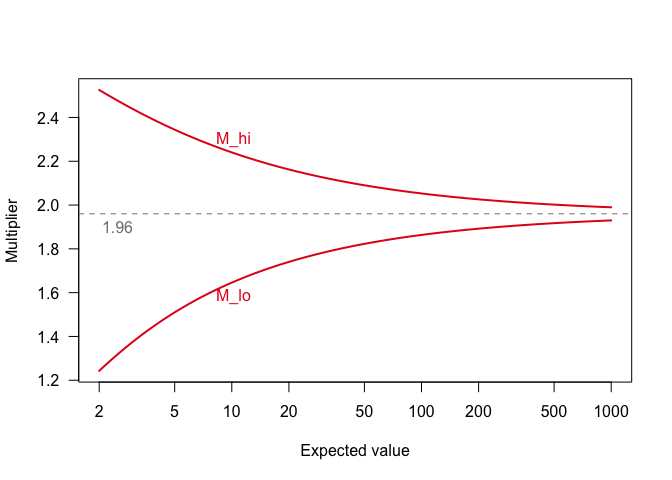Here is an example of how these multipliers can be used to compute confidence intervals for TRIM time totals, using the Skylark dataset, model 3 (independent year effects) and no overdispersion (i.e. Poisson-type variance assumptions).

library(rtrim)
# Run basic TRIM model without overdispersion
data(skylark2)
m <- trim(count ~ site + year, data=skylark2, model=3, overdisp=FALSE)
tt <- totals(m)

alpha <- 0.05 # define confidence interval: 95%
lambda <- tt$imputed # use imputed time totals as expected value qlo <- qgamma(p=alpha/2, shape=lambda) # Compute multipliers qhi <- qgamma(p=1-alpha/2, shape=lambda) lmul <- (lambda-qlo) / sqrt(lambda) umul <- (qhi-lambda) / sqrt(lambda) tt$lo <- tt$imputed - tt$se_imp * lmul # Compute CI bounds
tt$hi <- tt$imputed + tt$se_imp * umul plot(tt) lines(tt$time, tt$lo, lty="dashed", col=red) lines(tt$time, tt\$hi, lty="dashed", col=red)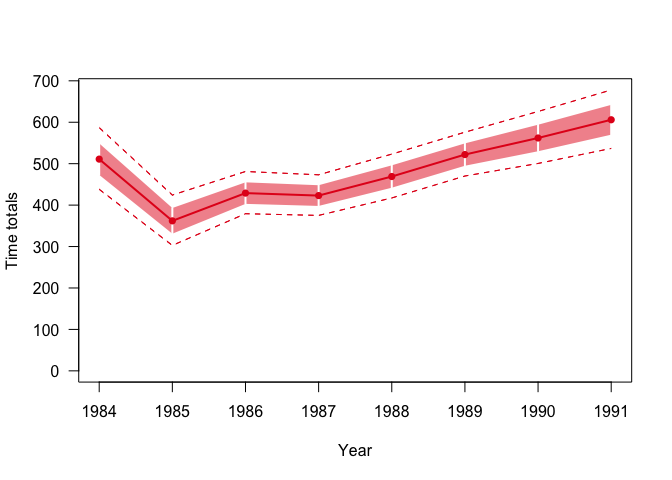In fact, the computation of confidence intervals is now built into R-TRIM, using the level argument for totals(). The resulting columns with lower and upper confidence bounds are drawn by plot() automatically.

tt <- totals(m, level=0.95)
plot(tt)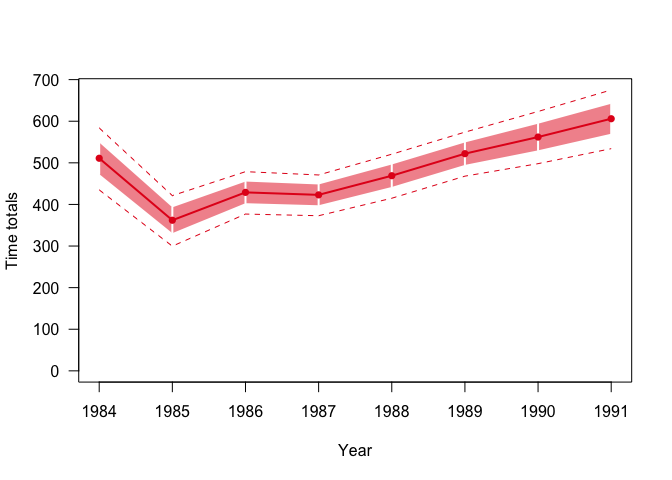By setting the band="ci" option in plot(), the uncertainty band is drawn using the confidence intervals, rather than the standard errors.

plot(tt, band="ci")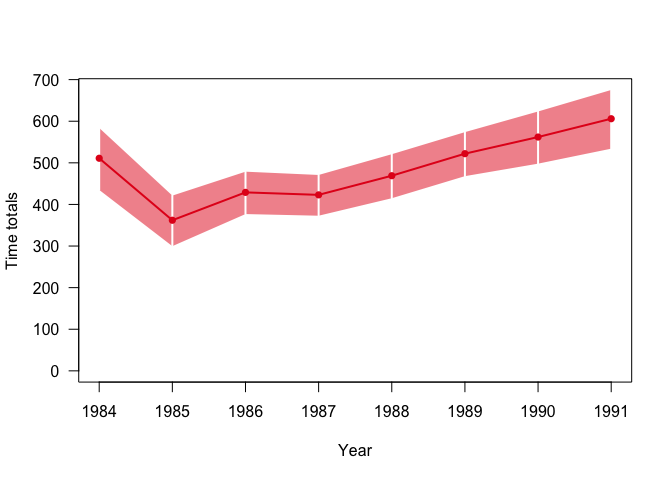# Taking overdispersion into account

One of the relaxations of TRIM with respect to the Poission regression assumptions is that variance does not need to equal variance, but instead is allowed to be proportionally larger, i.e. $$\operatorname{var}[x] = \sigma^2 \operatorname{E}[x]$$, where $$\sigma^2$$ is an overdispersion parameter, which is a scalar parameter estimated by TRIM.

This alternative constraint on variance is easily expressed using a negative binomial distribution $$\operatorname{NB}(r,p)$$ where $$r$$ is a parameter and $$p$$ is a . In ecological applications an alternative parameterization is often used, using $$r$$ and mean $$\mu$$. In this case, the first two moments are given by $\operatorname{E}[x] = \mu$ and $\operatorname{var}[x] = \mu + \frac{\mu^2}{r}$ Using the overdispersion constraint $$\operatorname{var}[x] \equiv \sigma^2 \operatorname{E}[x]$$ we get $\mu + \frac{\mu^2}{r} \equiv \sigma^2 \mu$ $\Rightarrow 1 + \frac{\mu}{r} = \sigma^2$ $\Rightarrow r = \frac{\mu}{\sigma^2-1}$ Here is an example showing the CDF’s for $$\sigma^2=1$$ (Poisson) and $$\sigma^2=2,5,10$$, using $$\mu=\lambda=100$$.

mu <- 100L
sig2 <- c(1, 2, 5, 10)
n <- length(sig2)
colors <- c("#E41A1C","#377EB8","#4DAF4A","#984EA3") # ColorBrewer "Set1" colors 1..4
x <- seq.int(0, 2*mu, by=2)
plot(NULL, NULL, type="n", xlim=range(x), ylim=c(0,1),
xlab="Value", ylab="Cumulative distribution function", las=1)
for (i in 1:n) {
y <- if (sig2[i]==1) ppois(x, mu)
else            pnbinom(x, mu=mu, size=mu/(sig2[i]-1))
points(x, y, col=colors[i], pch=16, cex=0.5)
}
# mark expected value
abline(v=mu, col=mgray, lty="dashed")
text(mu, 0.1, expression(lambda), pos=4, col=mgray)
leg_msg <- sprintf("Overdispersion = %d", sig2)
leg_msg <- "Poisson"
legend("topleft", legend=leg_msg, col=colors[1:n], pch=16)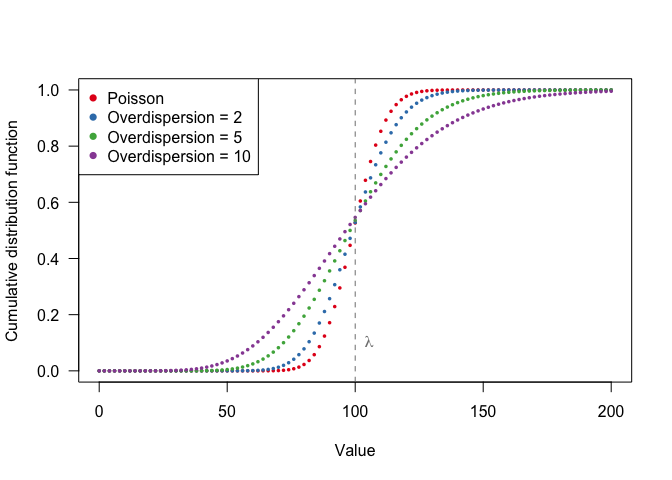As with the Poisson distribution, the Negative Binomial distribution can well be approximated by a Gamma distribution. Again, we use the methods of equal moments to find the Gamma parameters $$k,\theta$$ corresponding to the relaxed assumptions $$\operatorname{var}[x] = \sigma^2 \operatorname{E}[x] = \sigma^2\mu = \sigma^2\lambda$$: $\operatorname{E}[x] = k\theta = \mu \Rightarrow \theta = \frac{\mu}{k}$ $\operatorname{var}[x] = \sigma^2\mu \Rightarrow k \theta^2 = \sigma^2\mu$ $\Rightarrow k \left(\frac{\mu}{k}\right)^2 = \sigma^2 \mu$ $\Rightarrow k = \frac{\mu}{\sigma^2}$ Going back to our expression for $$\theta$$: $\theta = \frac{\mu}{k} \Rightarrow \theta=\sigma^2$

Here is the previous example of four different overdispersion parameters, for both the Poisson / Negative Binomial distibutions enhanced by plotting the corresponding Gamma distributions.

plot(NULL, NULL, type="n", xlim=range(x), ylim=c(0,1),
xlab="", ylab="Cumulative distribution function", las=1)
# Plot discrete distributions: Poisson or Negative Binonmial
for (i in 1:n) {
y <- if (sig2[i]==1) ppois(x, mu)
else            pnbinom(x, mu=mu, size=mu/(sig2[i]-1))
points(x, y, col=colors[i], pch=16, cex=0.5)
}
# Plot continuous distributions: Gamma
for (i in 1:n) {
y <- pgamma(x, shape=mu/sig2[i], scale=sig2[i])
lines(x, y, col=colors[i])
}
# mark expected value
abline(v=mu, col=mgray, lty="dashed")
text(mu, 0.1, expression(lambda), pos=4)
leg_msg <- sprintf("Overdispersion = %d", sig2)
leg_msg <- "Poisson"
legend("topleft", legend=leg_msg, col=colors[1:n], pch=16)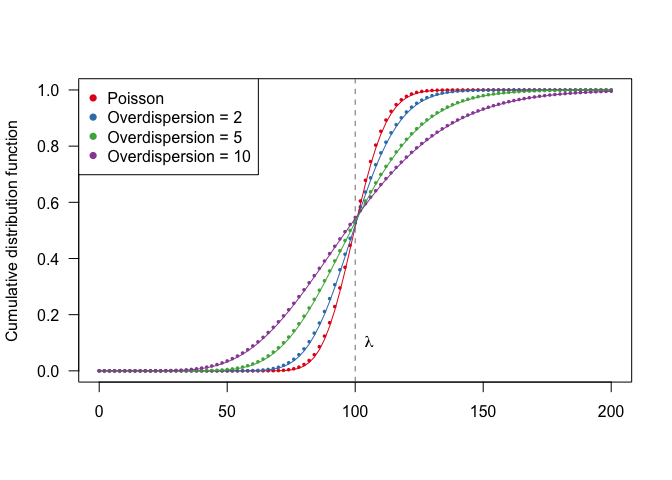showing again the acceptable degree of approximation.

## Multipliers

Multipliers relating standard errors with confidence interval bounds can be computed using the same approach as demonstrated above for the Poisson distribution. Here is an example for $$\sigma^2 = 1 \ldots 10$$

lambda <- exp(seq(log(2.0), log(1000.0), len=100)) # uniform spacing in log-space
alpha <- 0.05 # 95% CI
sig2  <- c(1, 2, 5, 10)
n <- length(sig2)
colors <- c("#E41A1C","#377EB8","#4DAF4A","#984EA3") # ColorBrewer "Set1" colors 1..4

plot(NULL, NULL, xlim=range(lambda), ylim=c(0,3), log="x",
xlab="Expected value", ylab="Multiplier", las=1)

for (i in 1:n) {
qhi <- qgamma(p=1-alpha/2, shape=lambda/sig2[i], scale=sig2[i])
qlo <- qgamma(p=alpha/2,   shape=lambda/sig2[i], scale=sig2[i])
sd <- sqrt(sig2[i] * lambda)
umul <- (qhi-lambda) / sd
lmul <- (lambda-qlo) / sd
lines(lambda, umul, col=colors[i], lwd=2)
lines(lambda, lmul, col=colors[i], lwd=2)
# idx <- which(lambda>=10)
# text(lambda[idx],umul[idx],"M_hi", pos=3, col=red)
# text(lambda[idx],lmul[idx],"M_lo", pos=1, col=red)\
}
m0 <- qnorm(0.975) # Multiplier for normal distibutions, i.e. 1.96
abline(h=m0, col=mgray, lty="dashed", lwd=2)
text(2.5,m0,sprintf("%.2f",m0), col=mgray, pos=1)
leg_msg <- sprintf("Overdispersion = %d", sig2)
leg_msg <- "Poisson"
legend("bottomright", legend=leg_msg, col=colors[1:n], pch=16)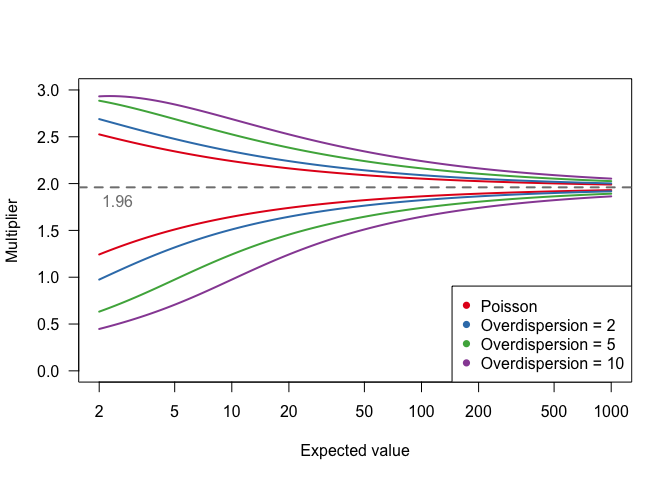Note that while upper-bound multipliers increase with overdispersion, lower-bound multipliers decrease. This is easily understood from the skewness of these distributions and the constraints that the lower bound is constrained to be $$>0$$. The following plot illustrates the principle for $$\lambda=20$$: and $$\sigma^2=8$$

lambda <- 20
sig2   <- 8
alpha <- 0.05 # 95% CI
n <- length(sig2)

# Discrete: Poisson & Neg. Binomial
x <- 0 : (3*lambda)
y <- dnbinom(x, mu=lambda, size=lambda/(sig2-1))
plot(x,y, xlab="Value", ylab="Frequency", type='s', col=red, las=1)
points(x, y, col=red, pch=16, cex=0.5)

# Expected value as solid vertical line
abline(v=lambda, col=mgray, lwd=2)
# Standard errors as dashed lines
sd <- sqrt(sig2 * lambda)
abline(v=c(lambda-sd,lambda+sd), lty="dashed", col=blue, lwd=2)
# plot CI intervals as dotted lines
lo <- qgamma(p=  alpha/2, shape=lambda/sig2, scale=sig2)
hi <- qgamma(p=1-alpha/2, shape=lambda/sig2, scale=sig2)
abline(v=c(lo,hi), lty="dotted", col=green, lwd=2)
legend("topright", c("mean","std.err","C.I."), lty=c("solid","dashed","dotted"),
lwd=2, col=c(mgray,blue,green))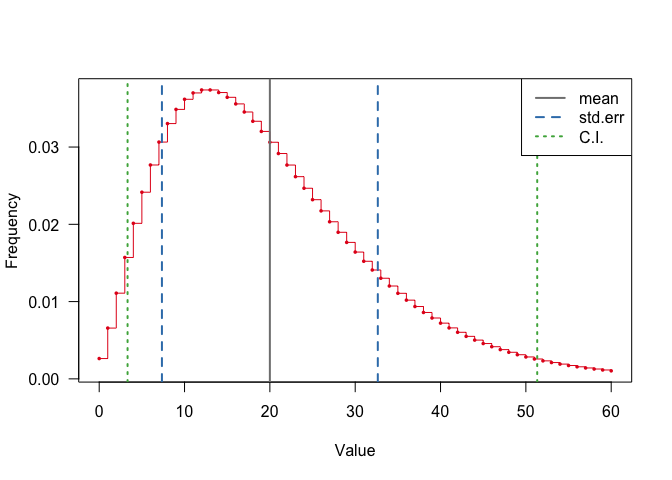Things change when $$\sigma^2 > \lambda$$, in this case the standard errors are larger than the mean, such that the multipliers must be $$<1$$ to ensure the confidence interval be positive. Here is an example for $$\lambda=10$$ and $$\sigma^2=12$$

lambda <- 10
sig2   <- 12
alpha <- 0.05 # 95% CI
n <- length(sig2)

# Discrete: Poisson & Neg. Binomial
x <- 0 : (3*lambda)
y <- dnbinom(x, mu=lambda, size=lambda/(sig2-1))
plot(x,y, xlab="Value", ylab="Frequency", type='s', col=red, las=1)
points(x, y, col=red, pch=16, cex=0.5)

# Expected value as solid vertical line
abline(v=lambda, col=mgray, lwd=2)
# Standard errors as dashed lines
sd <- sqrt(sig2 * lambda)
abline(v=c(lambda-sd,lambda+sd), lty="dashed", col=blue, lwd=2)
# plot CI intervals as dotted lines
lo <- qgamma(p=  alpha/2, shape=lambda/sig2, scale=sig2)
hi <- qgamma(p=1-alpha/2, shape=lambda/sig2, scale=sig2)
abline(v=c(lo,hi), lty="dotted", col=green, lwd=2)
legend("topright", c("mean","std.err","C.I."), lty=c("solid","dashed","dotted"),
lwd=2, col=c(mgray,blue,green))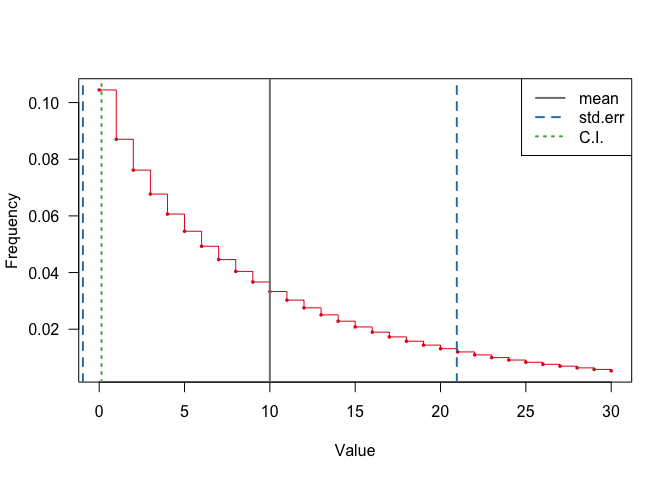It is recommended that these cases (i.e. $$\sigma^2 > \lambda$$) be avoided when fitting rtrim models.

The folowing diagram shows the multipliers for a range of overdispersion parameters, indicating the domain of applicability (i.e. $$\sigma^2 < \lambda$$)

lambda <- exp(seq(log(2.0), log(1000.0), len=100)) # uniform spacing in log-space
alpha <- 0.05 # 95% CI
sig2  <- c(1, 3, 10, 30, 100)
n <- length(sig2)
colors <- c("#E41A1C","#377EB8","#4DAF4A","#984EA3","#FF7F00") # ColorBrewer "Set1" colors 1..5

plot(NULL, NULL, xlim=range(lambda), ylim=c(0,3), log="x",
xlab="Expected value", ylab="Multiplier")

for (i in 1:n) {
qhi <- qgamma(p=1-alpha/2, shape=lambda/sig2[i], scale=sig2[i])
qlo <- qgamma(p=alpha/2,   shape=lambda/sig2[i], scale=sig2[i])
sd <- sqrt(sig2[i] * lambda)
umul <- (qhi-lambda) / sd
lmul <- (lambda-qlo) / sd
lines(lambda, umul, col=colors[i], lwd=1, lty="dashed")
lines(lambda, lmul, col=colors[i], lwd=1, lty="dashed")
# plot feasible range
ok <- lambda > sig2[i]
lines(lambda[ok], umul[ok], col=colors[i], lwd=2)
lines(lambda[ok], lmul[ok], col=colors[i], lwd=2)
}
abline(h=qnorm(0.975), col=mgray, lty="dashed", lwd=2)
leg_msg <- sprintf("Overdispersion = %d", sig2)
leg_msg <- "Poisson"
legend("bottomright", legend=leg_msg, col=colors[1:n], lty="solid")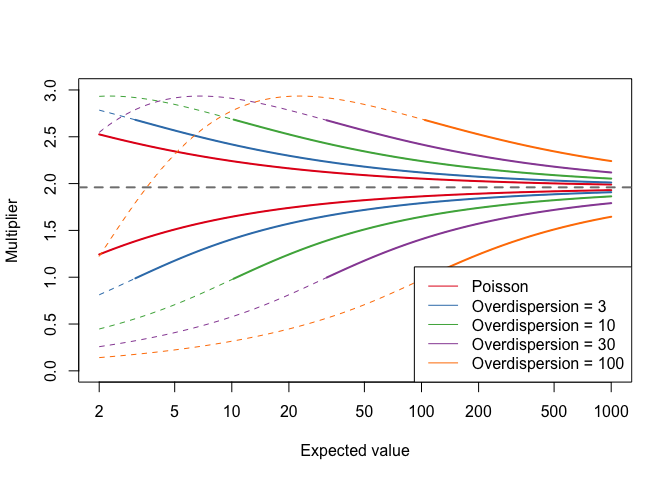Note that because of our feasibility constraints all mulitplier–expected value relations look similar now, expcept for the obvious shift to the right.

Again, these general-case multipliers (i.e. both Poisson and Overdispersion assumptions) can be applied to the Skylark example to create and plot confidence intervals.

# Run TRIM with overdispersion
m1 <- trim(count ~ site + year, data=skylark2, model=3, overdisp=TRUE)
tt1 <- totals(m1, level=0.95)
plot(tt1, main=sprintf("Skylark; overdispersion=%.2f", overdispersion(m1)))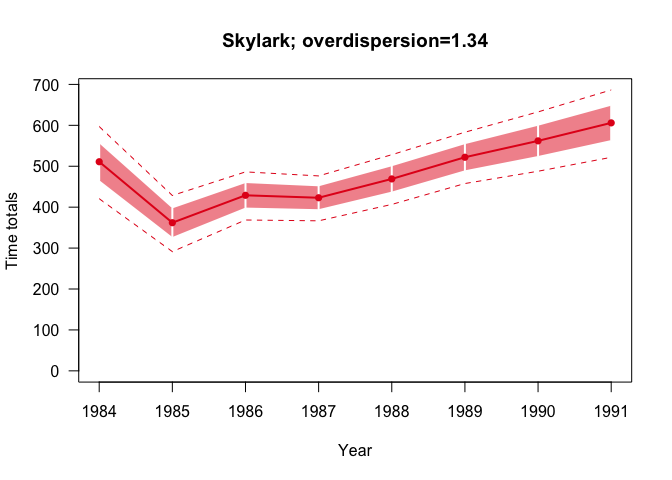In this case, the effect of overdispersion is very limited. For an example where overdispersion is large, see the vignettes Taming overdispersion and [rtrim 2.0 extensions](rtrim_2_extensions.html

# Confidence intervals for indices

Finally, an example of how confidence intervals are computed and plotted for indices as well:

idx <- index(m1, level=0.95)
plot(idx, pct=TRUE)`